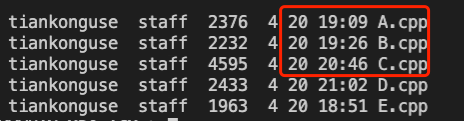# 2021 TPC 腾讯程序设计竞赛（2）

## 零、背景

2021 年 TPC 腾讯程序设计竞赛 又开始了。

4 月 20 号，周二晚上，参加了 2021 年 TPC 腾讯程序设计竞赛第一季第二场比赛。

PS：这次在工位上作比赛。

## 一、奇怪的排序

``````int base;
int vec;

bool check(int n) {
for (int i = 1; i < n; i++) {
if (base[i] < base[i - 1]) {
return false;
}
}
return true;
}

if (n % 2 == 1) {
printf("Yes\n");
continue;
}

int m = n / 2;
for (int i = 0; i < n; i++) {
vec[i % 2][i / 2] = base[i];
}

sort(vec, vec + m);
sort(vec, vec + m);

for (int k = 0; k < n; k += 2) {
for (int i = 0; i < 2; i++) {
base[k + i] = vec[i][k / 2];
}
}

if (check(n)) {
printf("Yes\n");
} else {
printf("No\n");
}
}
``````

## 二、零的个数

`a ~ a[n]` 所有数字或运算后，二进制中 0 的个数。

``````ll forceCal(int n, ll a, ll b) {
ll sum = a | b;
for (int i = 2; i <= n; i++) {
ll c = (a + b) % 1024;
sum |= c;
a = b, b = c;
}
return sum;
}

int ans = 0;
ll sum = forceCal(min(n, 20), a, b);
while (sum > 0) {
if (sum % 2 == 0) {
ans++;
}
sum /= 2;
}

printf("%d\n", ans);
``````

## 三、最小生成树

-）要求制定的几个顶点必须是叶子节点。
-）输入有自环
-）输入有重边## 四、可整除连续子序列PS：复旦大学的同事提供了另一种思路，不需要暴力 check，不过复杂度还是 `O(n log(n))`

``````vector<int> vec;
unordered_map<int, int> m;

bool OK(int v) {
if (m.count(v)) return true;

for (auto& p : m) {
int u = p.first;
if (u % v == 0 || v % u == 0) {
continue;
}
return false;
}
return true;
}

int ans = 1;

int l = 0, r = 1;
int num = 1;
m.clear();
m[vec]++;

while (l < n && r <= n) {
while (r < n && OK(vec[r])) {
num++;
m[vec[r]]++;
r++;
ans = max(ans, num);
}

if (r == n) {
break;
}

while (l < r && !OK(vec[r])) {
num--;
m[vec[l]]--;
if (m[vec[l]] == 0) {
m.erase(vec[l]);
}
l++;
}

num++;
m[vec[r]]++;
r++;
}

printf("%d\n", ans);
``````

## 五、排列矩阵

k 个矩阵排列之后，可以组成 `N * m`的大矩阵，这个大矩阵存在一个最大的全 1 子矩阵。

## 六、最后

PS：还学错了，一开始学的 SPFA 最短路。。。

《完》

-EOF-

QQ算法群：165531769（不止算法）

## 关注公众号,接收最新消息

1.## 关注小密圈,学习各种算法

1.